# Steepest descent, method of

(diff) ← Older revision | Latest revision (diff) | Newer revision → (diff)
Jump to: navigation, search

A special instance of the method of descent (cf. Descent, method of), when the directionof descent is chosen as the direction opposite to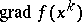. The formulas of the method of steepest descent arewhere the parametersare determined by the condition that the functionhas maximum decrease at each step. Ifis twice continuously-differentiable and its matrix of second derivativessatisfies the inequality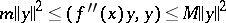for any, with constants, then (see , ) the sequence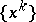converges to a solution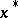of the problem of minimizing, the convergence rate being that of a geometric progression with quotient.

The method of steepest descent has been widely applied to the solution of systems of linear algebraic equationswith a Hermitian or positive-definite matrix. In the real symmetric case, the problem of solving this system is equivalent to finding a vectorin an-dimensional vector space minimizing the functional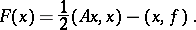(*)

Applied to (*), the method of steepest descent takes the form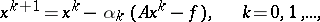where the value ofis determined by minimization of the functional (*), according to the formula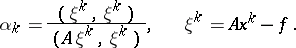If the spectrum oflies in an interval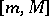,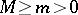, on the real axis, then the sequenceconverges to the solutionat the rate of a geometric progression with quotient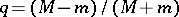.

The method of steepest descent can be applied to solve an operator equation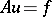with a self-adjoint positive-definite bounded operator. Ifdoes not satisfy these conditions, the problem can be symmetrized, reduced to the problemand then one can apply the method of steepest descent (see also Minimal discrepancy method).

How to Cite This Entry:
Steepest descent, method of. Encyclopedia of Mathematics. URL: http://encyclopediaofmath.org/index.php?title=Steepest_descent,_method_of&oldid=13529
This article was adapted from an original article by Yu.A. Kuznetsov (originator), which appeared in Encyclopedia of Mathematics - ISBN 1402006098. See original article